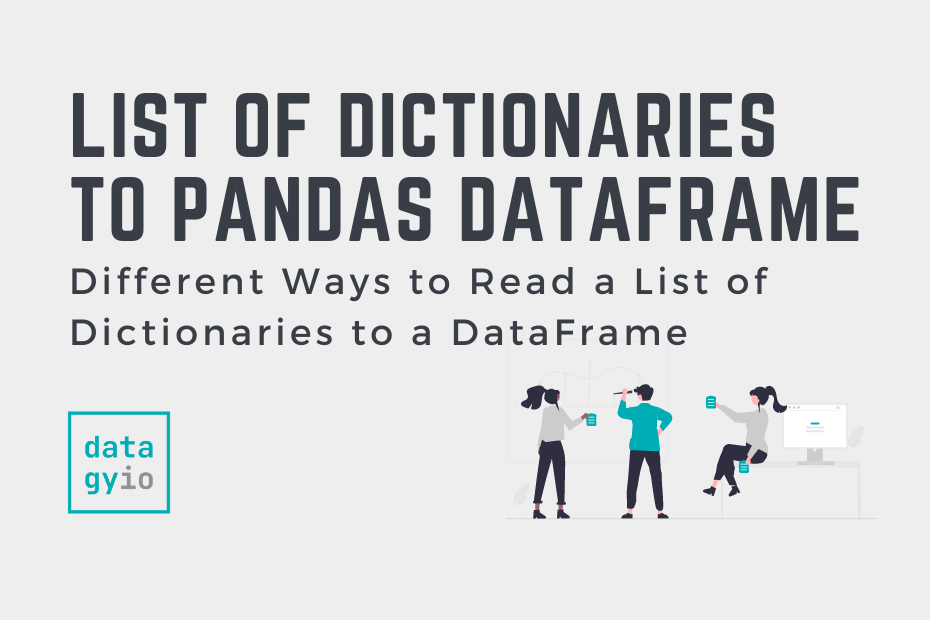# Pandas## Convert a List of Dictionaries to a Pandas DataFrame

In this tutorial, you’ll learn how to convert a list of Python dictionaries into a Pandas DataFrame. Pandas provides a number of different ways in which to convert dictionaries into a DataFrame. You’ll learn how to use the Pandas from_dict… Read More »Convert a List of Dictionaries to a Pandas DataFrame## How to Add / Insert a Row into a Pandas DataFrame

In this tutorial, you’ll learn how to add (or insert) a row into a Pandas DataFrame. You’ll learn how to add a single row, multiple rows, and at specific positions. You’ll also learn how to add a row using a… Read More »How to Add / Insert a Row into a Pandas DataFrame## Pandas Scatter Plot: How to Make a Scatter Plot in Pandas

In this tutorial, you’ll learn how to use Pandas to make a scatter plot. Under the hood, Pandas uses Matplotlib, which can make customizing your plot a familiar experience. Pandas allows you to customize your scatter plot by changing colors,… Read More »Pandas Scatter Plot: How to Make a Scatter Plot in Pandas## Pandas to_datetime: Convert a Pandas String Column to Date Time

In this tutorial, you’ll learn how to use the Pandas to_datetime function to convert a Pandas column to date time. Pandas provides a huge number of methods and functions that make working with dates incredibly versatile. However, data aren’t always… Read More »Pandas to_datetime: Convert a Pandas String Column to Date Time## K-Nearest Neighbor (KNN) Algorithm in Python

In this tutorial, you’ll learn how all you need to know about the K-Nearest Neighbor algorithm and how it works using Scikit-Learn in Python. The K-Nearest Neighbor algorithm in this tutorial will focus on classification problems, though many of the… Read More »K-Nearest Neighbor (KNN) Algorithm in Python## How to Calculate Mean Squared Error in Python

The mean squared error is a common way to measure the prediction accuracy of a model. In this tutorial, you’ll learn how to calculate the mean squared error in Python. You’ll start off by learning what the mean squared error… Read More »How to Calculate Mean Squared Error in Python## Combine Data in Pandas with merge, join, and concat

In this tutorial, you’ll learn how to combine data in Pandas by merging, joining, and concatenating DataFrames. You’ll learn how to perform database-style merging of DataFrames based on common columns or indices using the merge() function and the .join() method.… Read More »Combine Data in Pandas with merge, join, and concat## Splitting Your Dataset with Scitkit-Learn train_test_split

In this tutorial, you’ll learn how to split your Python dataset using Scikit-Learn’s train_test_split function. You’ll gain a strong understanding of the importance of splitting your data for machine learning to avoid underfitting or overfitting your models. You’ll also learn… Read More »Splitting Your Dataset with Scitkit-Learn train_test_split## Linear Regression in Scikit-Learn (sklearn): An Introduction

In this tutorial, you’ll learn how to learn the fundamentals of linear regression in Scikit-Learn. Throughout this tutorial, you’ll use an insurance dataset to predict the insurance charges that a client will accumulate, based on a number of different factors. You’ll… Read More »Linear Regression in Scikit-Learn (sklearn): An Introduction## Introduction to Random Forests in Scikit-Learn (sklearn)

In this tutorial, you’ll learn what random forests in Scikit-Learn are and how they can be used to classify data. Decision trees can be incredibly helpful and intuitive ways to classify data. However, they can also be prone to overfitting,… Read More »Introduction to Random Forests in Scikit-Learn (sklearn)## Introduction to Pandas for Data Science

In this tutorial, you’ll learn how to dive into the wonderful world of Pandas. Pandas is a Python package that provides fast and flexible data structures used for data manipulation and analysis. By the end of this tutorial, you’ll have… Read More »Introduction to Pandas for Data Science## Indexing, Selecting, and Assigning Data in Pandas

In this tutorial, you’ll learn how to index, select and assign data in a Pandas DataFrame. Understanding how to index and select data is an important first step in almost any exploratory work you’ll take on in data science. Similarly,… Read More »Indexing, Selecting, and Assigning Data in Pandas